# A conservation-law view of everyday motion

This plot of kinetic energy versus momentum has a place for most moving objects that folks encounter in everyday life. It shows objects with the same kinetic energy (horizontally related) that carry different amounts of momentum, as well as how the speed of a low-mass object compares (by vertical extrapolation) to the speed after perfectly inelastic collision with a larger object at rest. Highly sloped lines (rise/run = 2) mark contours of constant mass, while lines of unit slope mark contours of constant speed. Parallel contours are separated by intervals of three orders of magnitude, so objects from small (radio frequency photons) to large (the observable universe) are included. The top and right axes are labeled in practical units, while the bottom and left axes show SI unit values of the quantities being graphed. The plot further illustrates where lightspeed, Planck's constant, and kT figure in.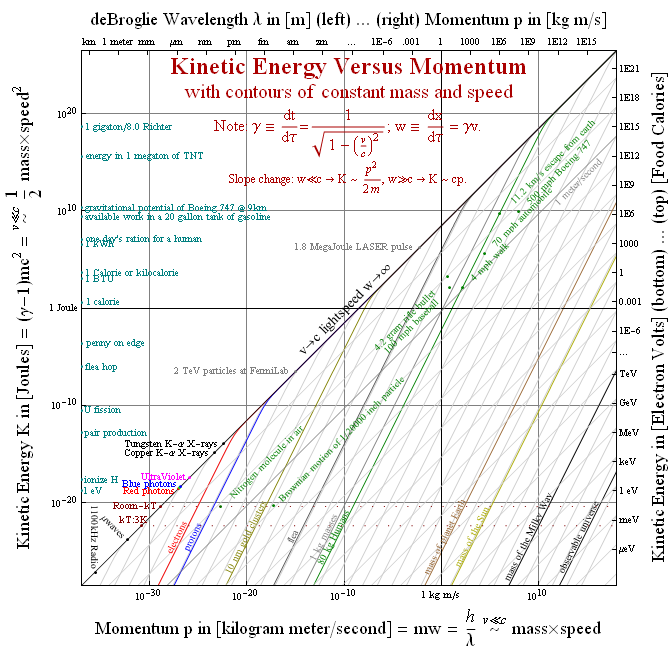Use this graph to quickly estimate the kinetic energy needed to accelerate an object to a given velocity (like a person to earth escape velocity), as well as the kinetic energy transferred in a momentum exchange between two objects of differing mass (like when you hit the planet after falling off the roof). It's also easy to see, for example, that laser weapons with many times the kinetic energy of a speeding automobile have much less recoil e.g. than that of a rifle.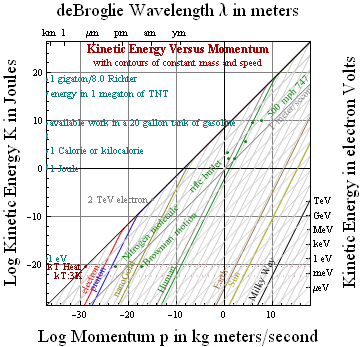The centered diagonal-line running from lower left to upper right is a "curtain of finite lightspeed c" that Newton had no reason to expect. When the curtain is in place redirecting lines of constant mass asymptotically onto v=c, only the lower right half of the plot is accessible. For most of that accessible area Newton's approximations work pretty darn well. Although coordinate velocity v=dx/dt is a dead-end variable near its upper limit, proper velocity w=dx/dτ and momentum p=mw as well as relativistic energy γmc2 remain useful on both sides of w=c. The resulting slope-change in energy versus momentum is in practice verified every time someone examines electron-wavelength as a function of energy, e.g. using the transmission electron microscope at your neighborhood hospital. Note that the location of that electron elbow is determined by the metric conversion factor c between meters and seconds, which also limits the rate at which photons can move.

Planck's constant h determines the deBroglie wavelength (useful for objects of low momentum), and kT yields the thermal energy: per nat of state uncertainty as well as per pair of quadratic modes for distributing heat. Thermal kinetic energy of translational motion in 3D is therefore about (3/2)kT per particle. Note that the relationship between the energy and momentum (also frequency and wavenumber) of an object is called its dispersion relation. Such relations can be plotted for non-vacuum media on this graph, thus illustrating for example the concepts of medium-conferred effective mass and frequency-dependent refractive index. For ring rotators of given density one can also superpose in the lower left corner of this plot the freeze-out zone due to angular momentum quantization, which requires that the rotator's deBroglie wavelength span the ring's circumference an integral number of times.

What are some other things that might be fun to plot on such a graph? A partial list begins here...

• A moving snail.
• Myosin molecule moving along an actin muscle fibril.
• 300 keV microscope electron.
• 1/refractive index dispersion profile for light in glass.
• A line for the mass of the observable universe.
• Recoil energy of the earth when you fall off the roof (down near kT).

What else?

One place to start might be Edwin Taylor's notes on the principle of least action, which also links to some papers by Tom Moore. These provide a foundation at all education levels for descriptions of motion based on the conservation laws for energy and momentum. On simplifying concepts the late William Shurcliff (1909-2006), who self-published a monograph on proper-velocity (w=dx/dτ here), said to me some years ago:

```I wish you had pointed out this neat fact: because proper speed can be infinite, improper speed is necessarily limited -- cannot exceed 3x108 m/sec. If someone asks: "Why is the speed of light limited?", we answer: "It is not limited if defined in a manner that requires no synchrony, i.e. if defined as a ratio of distance (defined most simply) and time duration (defined most simply)."```

# Show me the math!

Here find a draft list of equations for interconverting between an object's kinetic energy K, momentum p, rest mass m, coordinate speed (also group velocity) v=dx/dt, and deBroglie wavelength λ. In effect, these implement the free particle dispersion relation. Except for wavelength, the basic idea is to let you calculate any variable from any pair of other variables (like m,v) assuming that γ=dt/dτ and w=dx/dτ are defined as usual in terms of v above.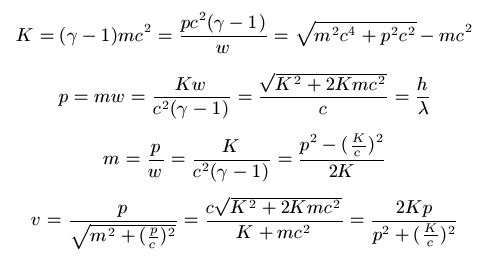Note also that when v is much less than c, proper velocity w is essentially equal to coordinate velocity v, and the kinetic energy per unit mass (γ-1)c2 becomes ½v2. The above relationships then simplify to their more familiar Newtonian forms: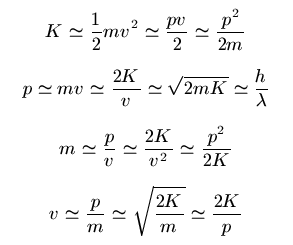Useful velocity-parameter conversions that work at any speed (at least in the uni-directional motion case) include: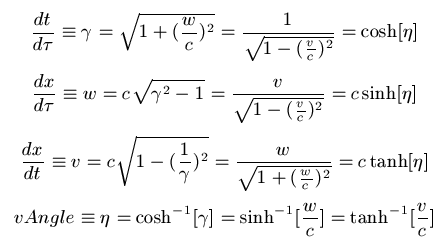These various velocity parameters are defined above in terms of the map-time t, map-position x, and proper-time τ variables used in the flat-space metric equation (cdτ)2 = (cdt)2 - (dx)2. That velocity angle (or rapidity) η comes in handy, for example, when considering the motion of accelerated objects. Of course, an integrative approach to anyspeed acceleration first starts with the v less than c case: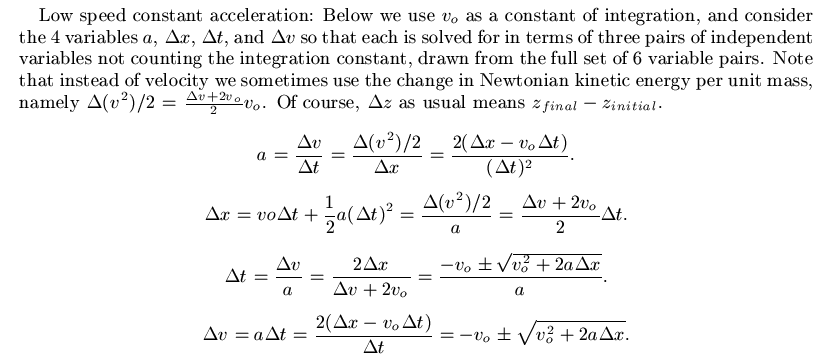This pattern of systematic solving can then be adapted for the anyspeed motion variables discussed above, if we replace the familiar coordinate acceleration a above with the proper acceleration α experienced by the accelerated object. Let us know if you find errors in these equations, as this is a first pass at putting them all in one place.Although we refer to the above as anyspeed equations, some of them may confuse your calculator at low speed because of roundoff errors in the high precision difference between two numbers. This is yet another reason that the simpler Newtonian equations come in handy for most of the accessible area in that kinetic energy versus momentum plot.

This site is hosted by the Department of Physics and Astronomy, and Center for NanoScience, at the University of Missouri in Saint Louis. Although there are many contributors, the person responsible for errors is P. Fraundorf. MindQuilts site page requests ~2000/day are approaching a million per year. Requests for a "stat-counter linked subset of pages" since 4/7/2005: .